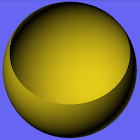All Android applications categories

All Android games categories# FuncXY

48 8.2

8.2 Users
rating

## Screenshots

Description

FuncXY is an application to plot 3D graphs of functions of two variables. In the simplest case, these are the Cartesian coordinates x and y. Version 2.00 introduced cylindrical coordinates theta and r as an alternative. Another extension, using spherical coordinates theta and phi, is also available in version 2.03. Finally, version 2.06 also supports parametric surfaces.

You can enter the formula for the function you want to plot. This must be an expression in two variables, for which you now have four options, depending on the type of coordinates:
Cartesian coordinates x and y, or
cylindrical coordinates theta and r, or
spherical coordinates theta and phi, or
parametric surfaces with coordinates u and v, in which not a single function but rather x, y and z are expressed.

You can use the arithmetical operators + - * / and ^ (for exponentiation). You can add parentheses in the usual way. Blank spaces are allowed. The minimum and maximum values of the two variables must be supplied. In the case of syntax errors or division by zero, the expression is not accepted. As you enter a formula, it is displayed in more readable notation just above the text you are entering or editing.

The function surface can be viewed from any viewpoint. There are buttons to change the viewpoint but you can also change the viewing direction by swiping the image, that is, by moving your finger on the screen either horizontally or vertically. You can also specify the viewing direction by entering two angles phiE and thetaE, as illustrated in the app itself.

In the 3D image, you can display or hide the coordinate axes as you like, by means of a checkbox on the choice screen. You can display this choice screen by using the back key.

The following functions, well-known in mathematics or programming, are supported:
abs, floor, ceil, exp, log, max, min, sin, cos, tan, asin, acos, atan, sqrt. You can also use the constant pi.
Arguments must be surrounded by parentheses. For example, sin(x), not sin x. As usual, max and min take two arguments, the others take one. Note the restricted domains of some of these functions:
log(x): x positive;
tan(x): x not equal to pi/2 (plus any multiple of pi);
asin(x), acos(x): x between -1 and +1 (inclusive).

For each of the four coordinate types, there are some example available in the options menu. When you choose an example, the required type of coordinates is chosen automatically, so you need not bother about this choice. Some of these examples show coordinate axes while other hide them. The checkbox on the choice screen enables you to change this.

The specified function can be saved and retrieved later. The following data are then also automatically saved and retrieved: the coordinate intervals, the viewpoint parameters phiE and thetaE, and the state of the checkbox for displaying the axes.

In version 2.07 and higher, conditional expressions are supported. These are well-known in programming languages and have the form "logical expression ? expression 1 : expression 2". For example, the expression max(x, y) could be written as the conditional expression: (x > y ? x : y). Besides "?" and ":", the following characters can now also be used: "<", ">", "=", "&", "", "1". You can use "=" or, as in real programming languages, "==" to test if two expressions are equal. Similarly, "&" and "" (meaning 'and' and 'or') can appear single or double, with the same meaning. The exclamation mark "!" stands for 'not'. Therefore, "x > y" can also be written "!(x <= y)". Similarly, "!(x == y)" has the same meaning as "x != y", both denoting 'x unequal to y'. The built-in example 2 illustrates a nested conditional expression, with the part following ":" containing another conditional expression.

Please refer also to
http://home.kpn.nl/ammeraal/funcxy.html

from 48 reviews

"Great"

8.2